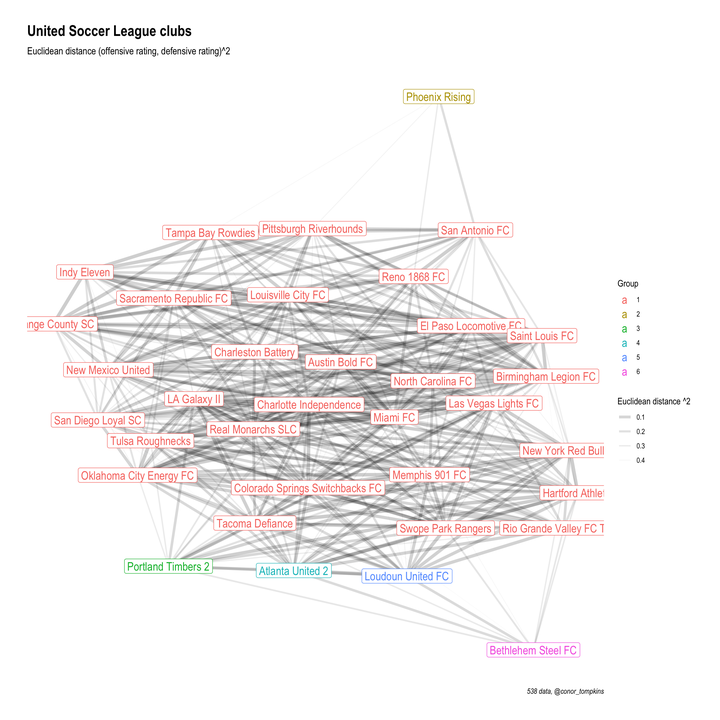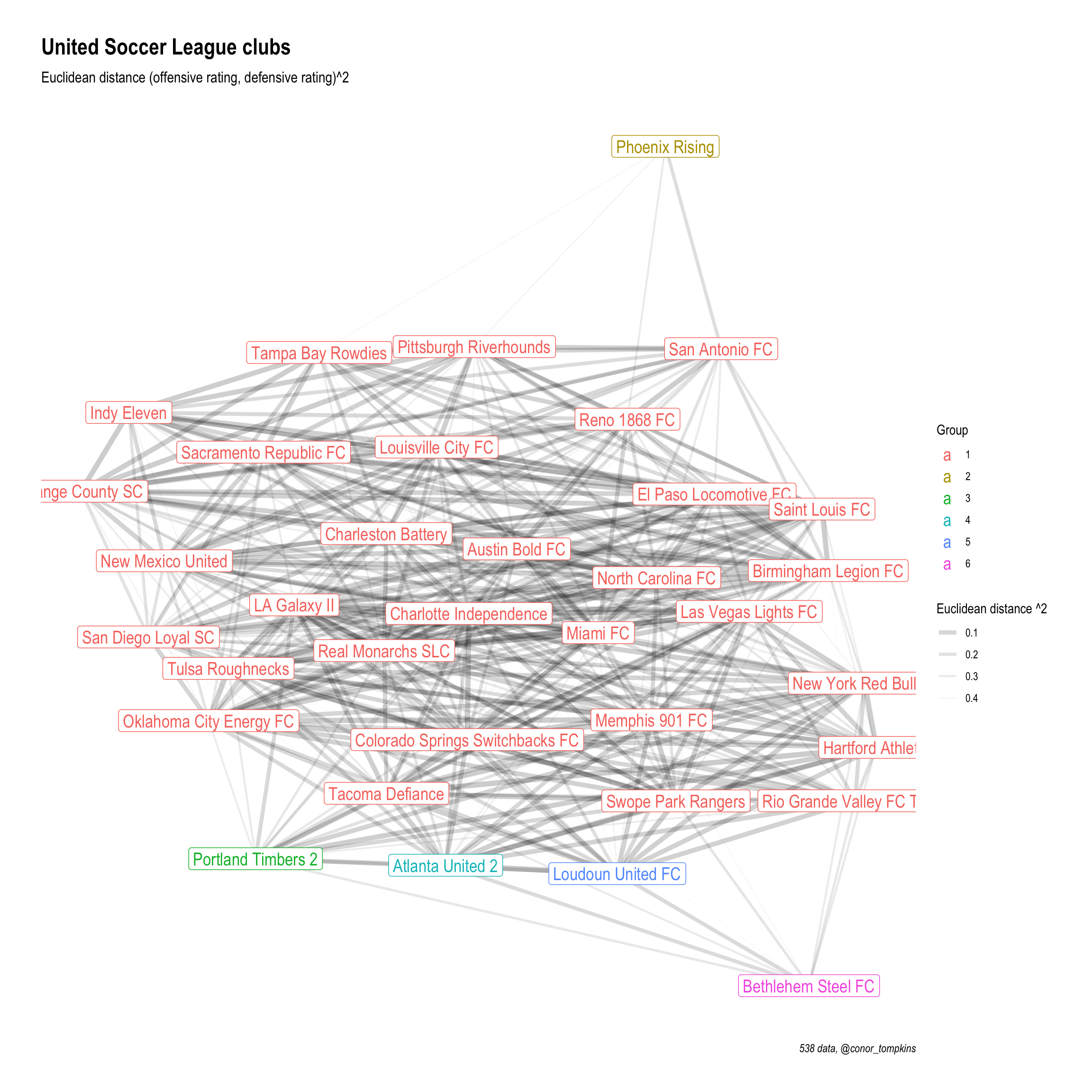# Networking USL Club Similarity With Euclidean DistanceEuclidean distance is a simple way to measure the distance between two points. It can also be used to measure how similar two sports teams are, given a set of variables. In this post, I use Euclidean distance to calculate the similarity between USL clubs and map that data to a network graph. I will use the 538 Soccer Power Index data to calculate the distance.

## Setup

``````library(tidyverse)
library(broom)
library(ggraph)
library(tidygraph)
library(viridis)

set_graph_style()

set.seed(1234)``````

## Download data

This code downloads the data from 538’s GitHub repo and does some light munging.

``````read_csv("https://projects.fivethirtyeight.com/soccer-api/club/spi_global_rankings.csv", progress = FALSE) %>%
filter(league == "United Soccer League") %>%
mutate(name = str_replace(name, "Arizona United", "Phoenix Rising")) -> df

df``````
``````## # A tibble: 35 x 7
##     rank prev_rank name                   league                 off   def   spi
##    <dbl>     <dbl> <chr>                  <chr>                <dbl> <dbl> <dbl>
##  1   263       257 Phoenix Rising         United Soccer League  1.59  1.77  42.4
##  2   419       428 San Antonio FC         United Soccer League  1.17  1.82  32.0
##  3   460       475 Pittsburgh Riverhounds United Soccer League  0.98  1.69  29.7
##  4   465       454 Tampa Bay Rowdies      United Soccer League  0.96  1.67  29.7
##  5   478       482 Reno 1868 FC           United Soccer League  1.06  1.92  27.9
##  6   498       496 Indy Eleven            United Soccer League  0.81  1.66  26.2
##  7   505       489 Orange County SC       United Soccer League  0.86  1.76  25.8
##  8   520       518 Louisville City FC     United Soccer League  0.85  1.84  24.2
##  9   533       528 New Mexico United      United Soccer League  0.9   2.01  22.9
## 10   534       532 Sacramento Republic FC United Soccer League  0.75  1.79  22.9
## # … with 25 more rows``````

## Calculate Euclidean distance

This is the code that measures the distance between the clubs. It uses the 538 offensive and defensive ratings.

``````df %>%
select(name, off, def) %>%
column_to_rownames(var = "name") -> df_dist

#df_dist
#rownames(df_dist) %>%
#  head()

df_dist <- dist(df_dist, "euclidean", upper = FALSE, diag = FALSE)
#head(df_dist)

df_dist %>%
tidy() %>%
arrange(desc(distance)) -> df_dist

#df_dist %>%
#  count(item1, sort = TRUE) %>%
#  ggplot(aes(item1, n)) +
#  geom_point() +
#  coord_flip() +
#  theme_bw()``````

## Network graph

In this snippet I set a threshhold for how similar clubs need to be to warrant a connection. Then I graph it using tidygraph and ggraph. Teams that are closer together on the graph are more similar. Darker and thicker lines indicate higher similarity.

``````distance_filter <- .5

df_dist %>%
mutate(distance = distance^2) %>%
filter(distance <= distance_filter) %>%
as_tbl_graph(directed = FALSE) %>%
mutate(community = as.factor(group_edge_betweenness())) %>%
ggraph(layout = "kk", maxiter = 1000) +
geom_edge_fan(aes(edge_alpha = distance, edge_width = distance)) +
geom_node_label(aes(label = name, color = community), size = 5) +
scale_color_discrete("Group") +
scale_edge_alpha_continuous("Euclidean distance ^2", range = c(.2, 0)) +
scale_edge_width_continuous("Euclidean distance ^2", range = c(2, 0)) +
labs(title = "United Soccer League clubs",
subtitle = "Euclidean distance (offensive rating, defensive rating)^2",
x = NULL,
y = NULL,
caption = "538 data, @conor_tompkins")``````